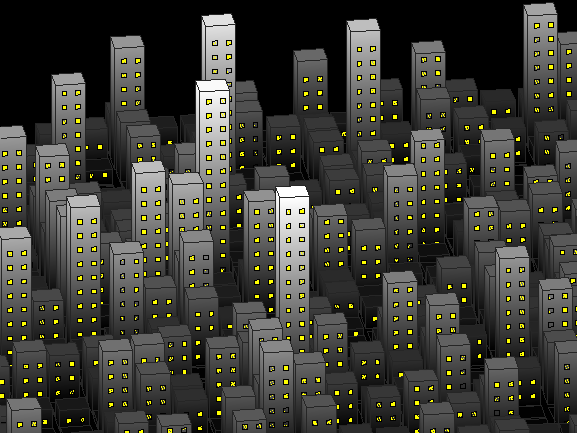# Life in the MATropolisAdam Danz on 10 Oct 2021
• 106
• 682
• 13
• 0
• 280
axes(colorm=gray,Vie=[172,22],Color='k')
hold
Current plot held
camva(3)
h=randg(1,25); % building heights
b=bar3(h);
axis equal
g=.13; % height and width of windows
[m,k]=ndgrid(1:25); % (x,y) building indices
for i=1:625 % loop through each building
% Interpolate building color according to building height
% Ideally this should be outside the loop but that would require more characters
q=b(m(i));
set(q,'CData',q.ZData,'FaceC','i')
for j=1:2 % loop through each column of windows
L=k(i)-.27+(j-1)*.37; % in k-a+(j-1)*b, a is the signed distance from center of building in row k(i) to left edge of window in column j and b is the interval between left edges of windows.
x=L+[0,g,g,0]'; % [L;R;R;L] edges of windows in building i, window column j
z=ones(4,1)*(.2:.4:h(i)-.2)+[0;0;g;g]; % 4xn height of windows for n levels of windows; in a:b:c a is height of first row of windows, b is the interval between window-bottoms, c is height of tallest window.
y=z*0+m(i)+.4; % 4xn location of front face of building
% Plot windows for building i, column j
patch(z*0+x,y,z,'y')
end
end